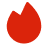# Twosigma计算机科学面试真题

BQ

Coding（4）
System Design（0）
Algorithm（0）
Ood（2）高频题（0）

Coding（4）
System Design（0）
Algorithm（0）
Ood（2）高频题（0）
1.LeetCode 297
2.Design sql
3.Design Currency conversion
4.LeetCode 509
5.LeetCode 706
6.missing word
7.股票竞价问题
8.leetcode1339
1. LeetCode 297
Serialization is the process of converting a data structure or object into a sequence of bits so that it can be stored in a file or memory buffer, or transmitted across a network connection link to be reconstructed later in the same or another computer environment.

Design an algorithm to serialize and deserialize a binary tree. There is no restriction on how your serialization/deserialization algorithm should work. You just need to ensure that a binary tree can be serialized to a string and this string can be deserialized to the original tree structure.

Clarification: The input/output format is the same as how LeetCode serializes a binary tree. You do not necessarily need to follow this format, so please be creative and come up with different approaches yourself.

Example 1:

```Input: root = [1,2,3,null,null,4,5]
Output: [1,2,3,null,null,4,5]
```
Example 2:

```Input: root = []
Output: []
```

Constraints:

• The number of nodes in the tree is in the range [0, 104].
• -1000 <= Node.val <= 1000
2. Design sql

3. Design Currency conversion
design currency conversion
4. LeetCode 509
The Fibonacci numbers, commonly denoted F(n) form a sequence, called the Fibonacci sequence, such that each number is the sum of the two preceding ones, starting from 0 and 1. That is,

```F(0) = 0, F(1) = 1
F(n) = F(n - 1) + F(n - 2), for n > 1.
```
Given n, calculate F(n).

Example 1:

```Input: n = 2
Output: 1
Explanation: F(2) = F(1) + F(0) = 1 + 0 = 1.
```
Example 2:

```Input: n = 3
Output: 2
Explanation: F(3) = F(2) + F(1) = 1 + 1 = 2.
```
Example 3:

```Input: n = 4
Output: 3
Explanation: F(4) = F(3) + F(2) = 2 + 1 = 3.
```

Constraints:

• 0 <= n <= 30
5. LeetCode 706
Design a HashMap without using any built-in hash table libraries.

Implement the MyHashMap class:

• MyHashMap() initializes the object with an empty map.
• void put(int key, int value) inserts a (key, value) pair into the HashMap. If the key already exists in the map, update the corresponding value.
• int get(int key) returns the value to which the specified key is mapped, or -1 if this map contains no mapping for the key.
• void remove(key) removes the key and its corresponding value if the map contains the mapping for the key.

Example 1:

```Input
["MyHashMap", "put", "put", "get", "get", "put", "get", "remove", "get"]
[[], [1, 1], [2, 2], , , [2, 1], , , ]
Output
[null, null, null, 1, -1, null, 1, null, -1]

Explanation
MyHashMap myHashMap = new MyHashMap();
myHashMap.put(1, 1); // The map is now [[1,1]]
myHashMap.put(2, 2); // The map is now [[1,1], [2,2]]
myHashMap.get(1);    // return 1, The map is now [[1,1], [2,2]]
myHashMap.get(3);    // return -1 (i.e., not found), The map is now [[1,1], [2,2]]
myHashMap.put(2, 1); // The map is now [[1,1], [2,1]] (i.e., update the existing value)
myHashMap.get(2);    // return 1, The map is now [[1,1], [2,1]]
myHashMap.remove(2); // remove the mapping for 2, The map is now [[1,1]]
myHashMap.get(2);    // return -1 (i.e., not found), The map is now [[1,1]]
```

Constraints:

• 0 <= key, value <= 106
• At most 104 calls will be made to put, get, and remove.
6. missing word
t is a subsequence of s,remove the first occurrence from s,and return the result
s = "I like soft cheese and hard cheese yum" t= "like cheese yum°
return" I soft and hard cheese"
7. 股票竞价问题